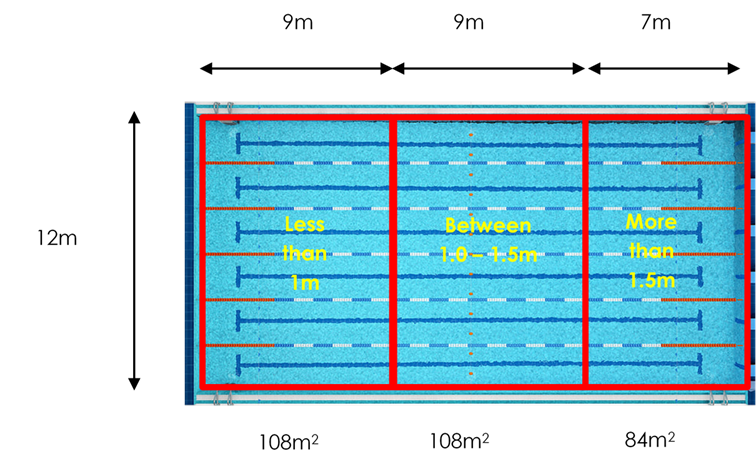It is important that pool operators do not over load a pool with bathers. It makes it difficult for lifeguards to spot people in trouble and it also has a negative impact in the quality of the pool water.

Divide the pool up into zones according to the depths given below:

• Less than 1.0 metre
• Between 1.0 – 1.5 metres
• More than 1.5 metres

Calculate the square meters of each zone by multiplying the length of the zone by the width of the zone.

Divide the square meters of each zone by the following figures:

• Less than 1.0 metre: divide by 2.2
• Between 1.0 – 1.5 metres: divide by 2.7
• More than 1.5 metres: divide by 4.0

Add the figures for each zone together to get an overall maximum instantaneous bathing load.

Example:• 108m2 divided by 2.2 = 49 (when rounded down)
• 108m2 divided by 2.7 = 40 (when rounded down)
• 84m2 divided by 4.0 = 21 (when rounded down)

49 + 40 + 21 = 110 would be the maximum instantaneous bathing load.